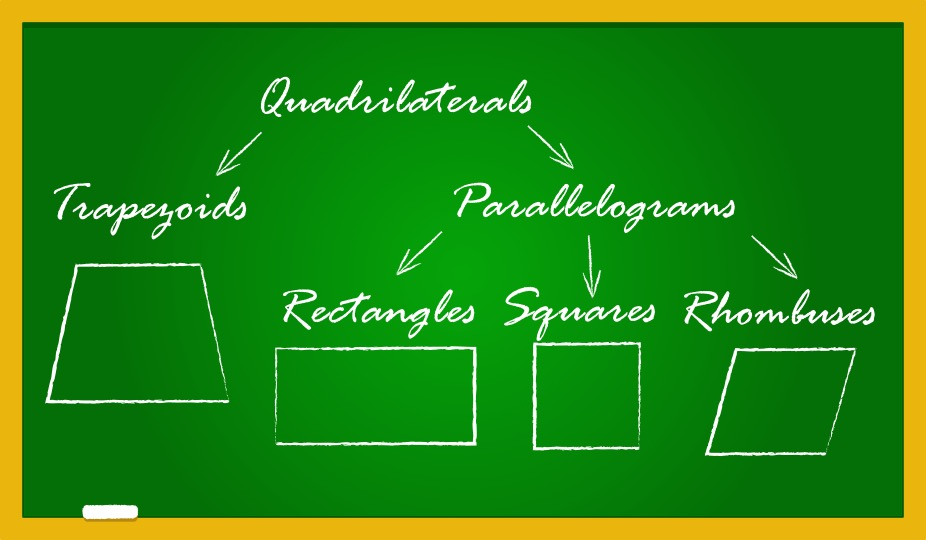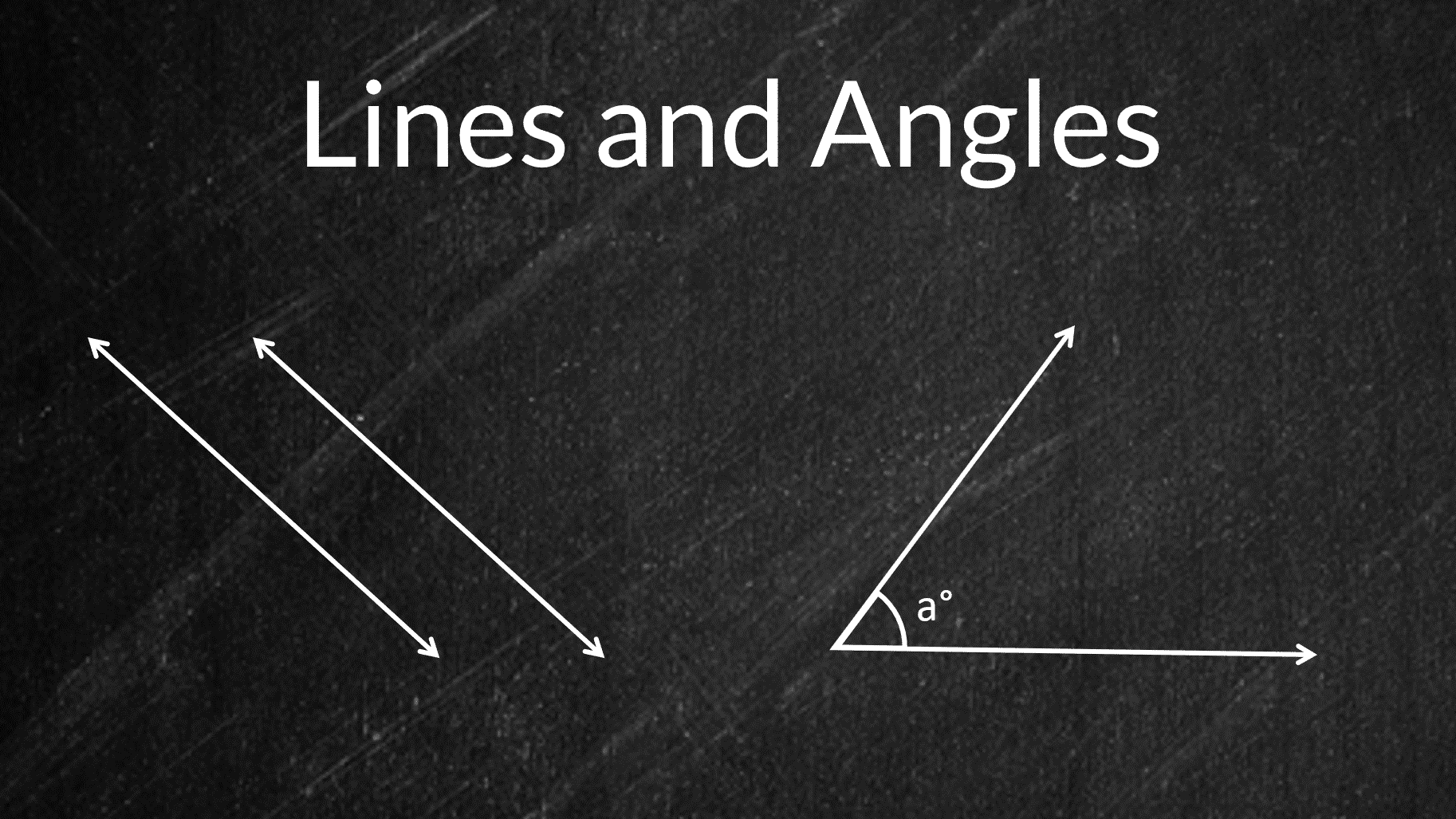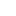Payal Tandon
Co-founder, e-GMAT
Welcome to e-GMAT Support!
I am Payal, Co-Founder of e-GMAT.
Feel free to ask any Query.We will be contacting you soon on

# If p, r, and s are consecutive integers in ascending order and x is the average (arithmetic mean) of the three integers – OG 2020 Question #304 with Solution

OG 2020: Question No. 304 If p, r, and s are consecutive integers in ascending order and x is the average (arithmetic mean) of the three integers, what is the value of x?...# Quadrilaterals – Properties of rectangle, square, parallelogram, rhombus, trapezium | 2023

In Euclidean geometry, a quadrilateral is a four-sided 2D figure whose sum of internal angles is 360°. The word quadrilateral is derived from two Latin words ‘quadri’ and...# What is a Circle and its properties? (Definition, Formulas, Examples)

A circle is a closed shape formed by tracing a point that moves in a plane such that its distance from a given point is constant. The word circle is derived from the Greek...

# If p, r, and s are consecutive integers in ascending order and x is the average (arithmetic mean) of the three integers – OG 2020 Question #304 with Solution

OG 2020: Question No. 304 If p, r, and s are consecutive integers in ascending order and x is the average (arithmetic mean) of the three integers,...# Quadrilaterals – Properties of rectangle, square, parallelogram, rhombus, trapezium | 2023

In Euclidean geometry, a quadrilateral is a four-sided 2D figure whose sum of internal angles is 360°. The word quadrilateral is derived from two...# What is a Circle and its properties? (Definition, Formulas, Examples)

A circle is a closed shape formed by tracing a point that moves in a plane such that its distance from a given point is constant. The word circle is...# Improve accuracy in GMAT Math questions on Polygons

A polygon is a two-dimensional closed figure made up of at least 3 line segments. Polygons, as a topic, will pose some of the most challenging GMAT...# Lines and Angles – Definitions, Properties, Types, Practice Questions

Ancient mathematicians introduced the concept of lines to represent straight objects which had negligible width and depth. Considered as a breadth...

# More articles

## If p, r, and s are consecutive integers in ascending order and x is the average (arithmetic mean) of the three integers – OG 2020 Question #304 with Solution

A 2 min read OG 2020: Question No. 304 If p, r, and s are consecutive integers in ascending order and x is the average (arithmetic mean) of the three integers, what is the value of x? Twice x is equal to the sum of p, r, and...

## GMAT Problem Solving – 3 mistakes you must avoid in Time and Work questions

A 10 min read GMAT problem solving questions on Time and Work can be solved easily and quickly provided you do not make mistakes while solving them. In the last article, we compared the conventional methods of solving Time and...

## GMAT Word Problems | Application of Average Speed in Time – Distance Problems

A 12 min read GMAT Word problems on Time, and Speed, and Distance are based on the simple formula of  D = S x T, where D is the distance, S is speed and T is time. These GMAT word problems might look easy at first but if...

## GMAT Data Sufficiency – 3 Mistake Students make in Number Properties questions

A 7 min read Typical GMAT data sufficiency questions are followed by information in the form of...

## How to get a Q51 on the GMAT

A 16 min read Among the many elements of your MBA application, the GMAT is the one that you have a...

## Properties of Numbers – Even & Odd | Prime | HCF & LCM

A 8 min read Hello Reader, We see that you want to know the basics and properties of numbers. You...

## How to quickly solve GMAT Quant Questions on Time and Work

A 13 min read Time and Work problems are the low-hanging fruit in the world of GMAT Quant...

## The dimensions of a ream of paper are 8 1/2 inches by 11 inches by 2 1/2 inches. The inside dimensions of a carton that will hold exactly 12 reams of paper could be – OGQR 2020 Question #93 with Solution

A 1 min read OGQR 2020: Question No. 93 The dimensions of a ream of paper are 8 1/2 inches by 11...

## If Car A took n hours to travel 2 miles and Car B took m hours to travel 3 miles, which of the following expresses the time it would take Car C, traveling at the average (arithmetic mean) of those rates, to travel 5 miles? – OGQR 2020 Question #79 with Solution

A < 1 min read OGQR 2020: Question No. 79 If Car A took n hours to travel 2 miles and Car B took m hours to travel 3 miles, which of the following expresses the time it would take Car C, traveling at the average (arithmetic...

## In the figure above, is the area of triangular region ADE equal to the area of rectangular region ABCD? – OGQR 2020 Question #206 with Solution

A 2 min read OGQR 2020: Question No.206 In the figure above, is the area of the triangular region ADE equal to the area of rectangular region ABCD? x = 10 and y = 5.x = 2y SourceOGQR 2020TypeData...###### Browse Related Topics# Achieve 740+on the GMAT in 30 days!Sign up for our free trial and get:400+ Practice questions with detailed solutions10+ hours of AI-driven video lessonsAdaptive mock test with ESR+ analysis